Home / Blog / Data Science Digital Book / Perceptron Algorithm

# Perceptron Algorithm

• July 15, 2023
• 4707
• 52### Meet the Author : Mr. Bharani Kumar

Bharani Kumar Depuru is a well known IT personality from Hyderabad. He is the Founder and Director of Innodatatics Pvt Ltd and 360DigiTMG. Bharani Kumar is an IIT and ISB alumni with more than 17 years of experience, he held prominent positions in the IT elites like HSBC, ITC Infotech, Infosys, and Deloitte. He is a prevalent IT consultant specializing in Industrial Revolution 4.0 implementation, Data Analytics practice setup, Artificial Intelligence, Big Data Analytics, Industrial IoT, Business Intelligence and Business Management. Bharani Kumar is also the chief trainer at 360DigiTMG with more than Ten years of experience and has been making the IT transition journey easy for his students. 360DigiTMG is at the forefront of delivering quality education, thereby bridging the gap between academia and industry.

The goal of artificial intelligence is to simulate the human brain.

An Artificial Neural Network (ANN) uses a model developed on our understanding of how a human brain responds to stimuli from sensory inputs to represent the relationship between a set of input signals and an output signal. An artificial neural network, or ANN, employs a network of artificial neurons or nodes to solve learning problems, much like a brain uses a network of linked cells called neurons to construct a large parallel processor.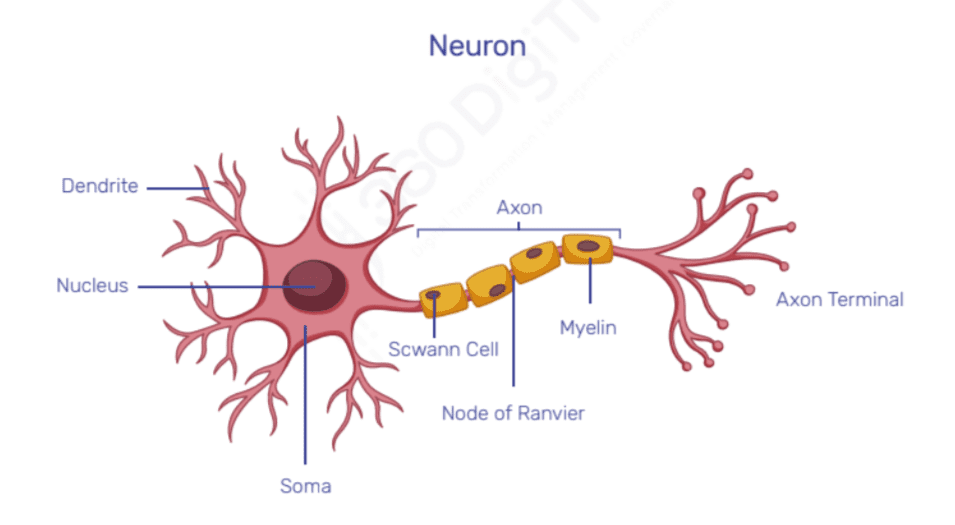### Simple Neural Network components:• Input layer - contains the numbers of neurons equal to the number of input features
• Input layer also has one additional neuron called bias, which is equivalent to the ‘b’ (y-intercept) in the equation of the line y = b + mx
• ‘b’, ‘w1’, ‘w2’, ‘w3’,....... are called as weights and are randomly initialized
• These neurons are also called as nodes and are connected via an edge to the neuron in the next layer
• Integration function (usually summation) is used to integrate all the inputs and corresponding weights f(x) = b + w1x1+ w2x2+ w3x3 + w4x4 + w5x5 This equation will give a numerical output
• The output of the integration function is passed on to the activation function component of the neuron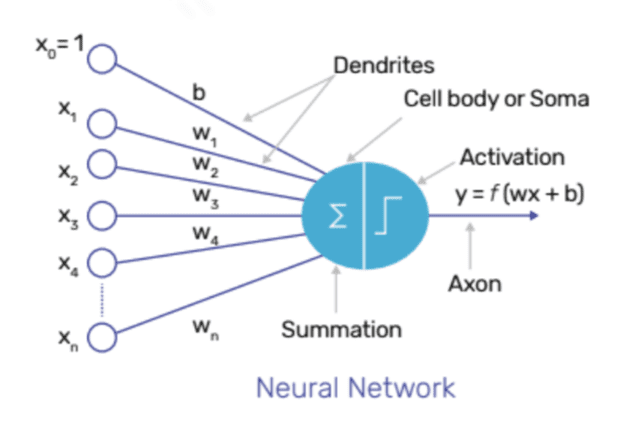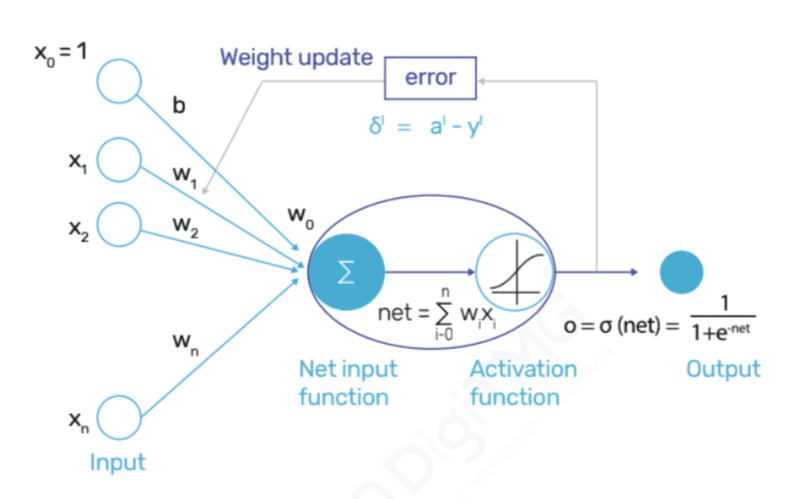• Based on the functioning of activation function, the final output is predicted
• Predicted output and actual output are compared to calculate the loss function / cost function (error calculated for each record is called as loss function and combination of all these individual errors is called as cost function)
• Based on this error, the backpropagation algorithm is used to go back in the network to update the weights
• Weights are updated with the objective of minimizing the error and this minimization of error is achieved using Gradient Descent Algorithm

### Perceptron Algorithm

Frank Rosenblatt of the Cornell Aeronautical Laboratory first introduced the Perceptron method in 1958.

A perceptron algorithm is a neural network with only one output neuron and no hidden layers.

Only linear boundaries can be handled by the Perceptron method. The Multi-Layered Perceptron technique is used to manage non-linear boundaries.

The backpropagation algorithm's weight updating is done using the following formula: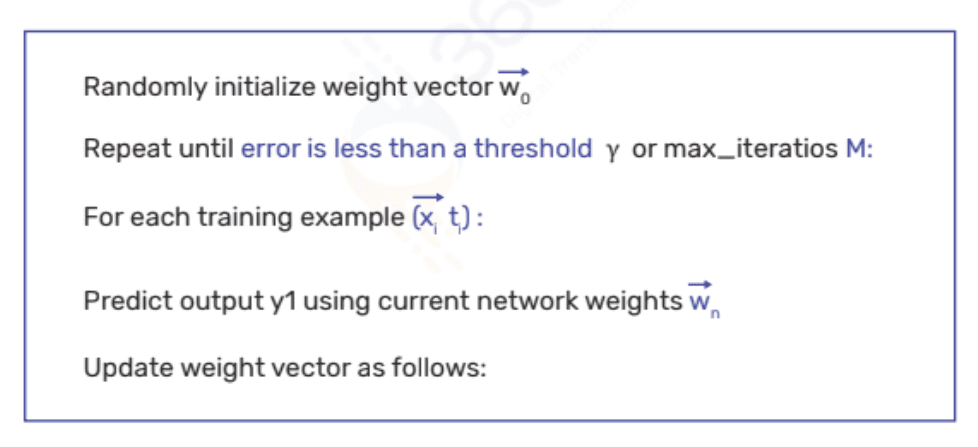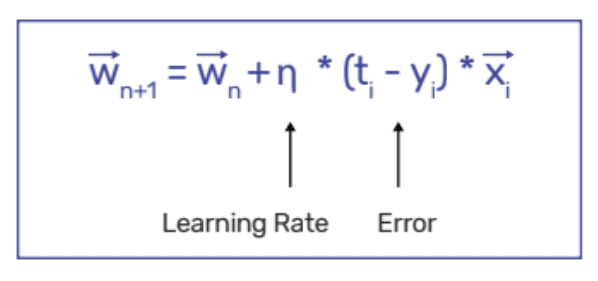In order to reduce mistake, weights are updated.

The range of the learning rate, often known as the eta value, is 0 to 1.

Infinite steps would be required to reach the bottom of the error surface if the value was near to 0.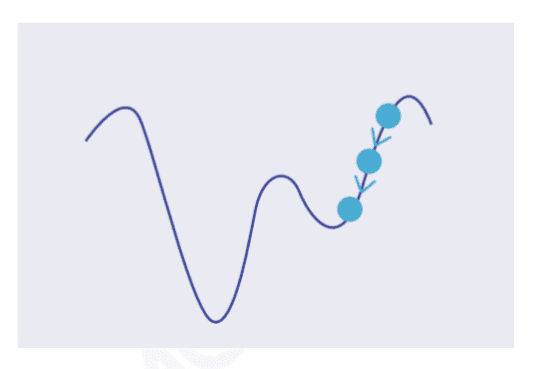A number around 1 would indicate overshooting the error surface's bottom.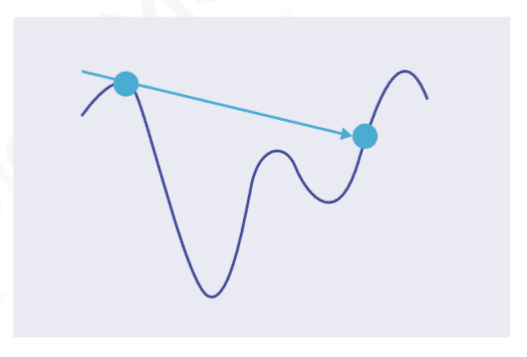The issue of bouncing around the bowl is brought on the constant learning rate.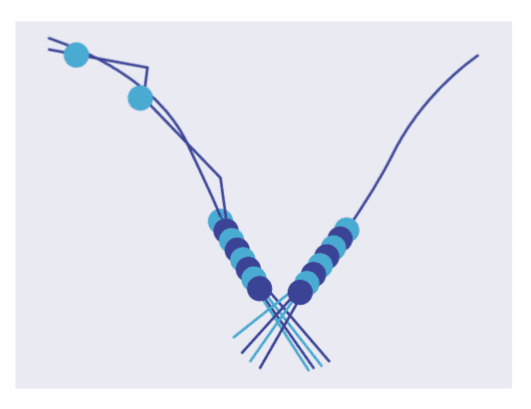The gradient will never touch the error surface's bottom.Changing Learning Rate (Shrinking Learning Rate) is used to tackle this issue.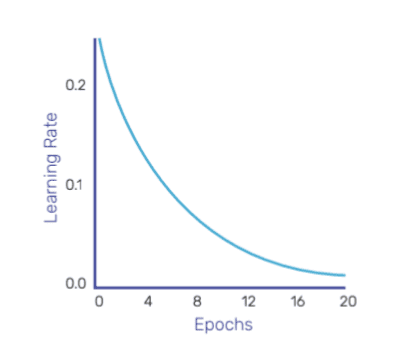Exponential Decay: The learning rate decreases epoch by epoch until a certain number of epochs have passed.Delayed Exponential Decay: The learning rate remains constant for a certain number of epochs, after which it starts to decline until the predetermined number of epochs is reached.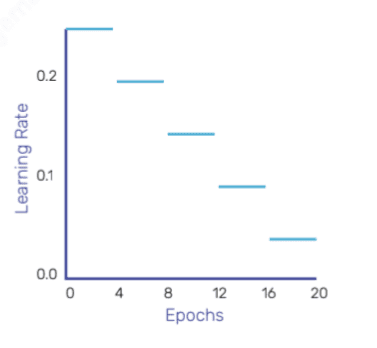Fixed-Step Decay: The learning rate is decreased after a predetermined number of epochs (for instance, the learning rate is decreased by 10% every five epochs).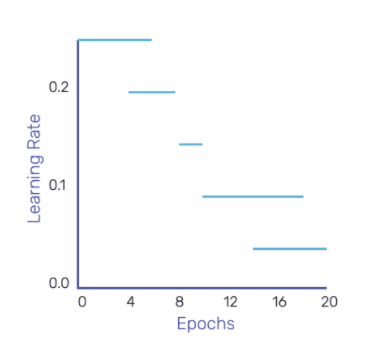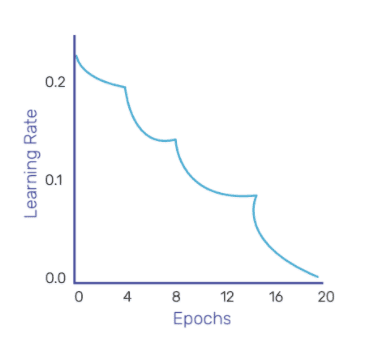When it is seen that the mistake is no longer decreasing, the learning rate is lowered.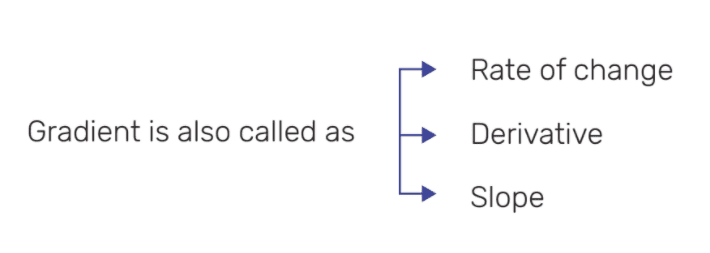Curves / Surfaces should be continuous and smooth (no cusps / sharp points)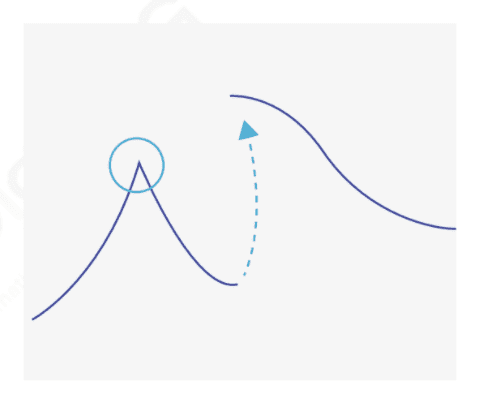Curves / Surfaces should be single-valued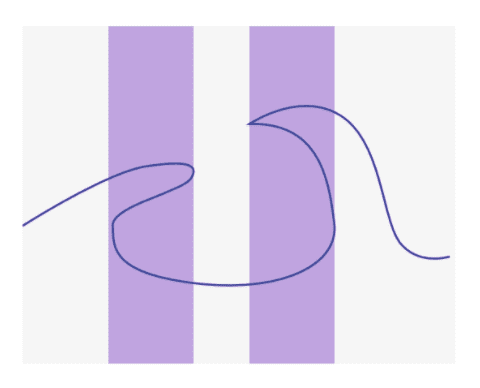A few definitions:

Iteration: Equivalent to when a weight update is done

Epoch: When entire training set is used once to update the weights

Epoch 1 1 1
Example 10000 training records 10000 training records 10000 training records
Iteration 1 10000 100 (if minibatch size is 100).
10000/100 = 100 iterations
Example Weights are updated once, after all 10000 training records are passed through the network Weights are updated after each training sample passes through the network. If we have 10000 training samples then weights are updated 10000 times Weights are updated after every minibatch (100 in this case) of records are passed through the network. Records within minibatch are randomly chosen.

Other advanced variants of Mini-Batch SGD: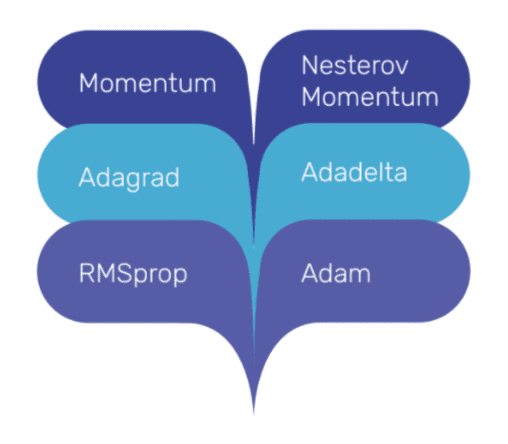### Empirically Determined components are:

• Number of hidden layers
• Number of neurons within each hidden layer
• Activation functions
• Error/Cost/Loss Functions
Y(output) No. of neurons in output layers Activation Function in Output layer Loss Function
Continuous 1 Linear / Identify ME, MAE, MSE, etc.
Discrete (2 categories) 1 for binary classification problem Sigmoid / Tanh Binary Cross Entropy
Discrete (>2 categories) 10 if we have a 10 class problem Sigmoid Categorical Cross Entropy

Note: Any activation function can be used in hidden layers, however ReLU activation functions often tend to provide positive outcomes.

Click here to learn Data Science Course, Data Science Course in Hyderabad, Data Science Course in Bangalore

### Data Science Training Institutes in Other Locations